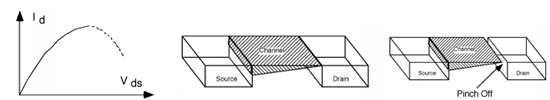## Equation for drain current Assignment Help

Assignment Help: >> Metal-Oxide-Semiconductor Field Effect Transistor - Equation for drain current

Equation for drain current:

Let us write down the equation for drain current

Id  =  (μs Cox  W )/L  (Vgs  - VT ) Vds  -  ( Vds )2 /2)

This equation is called the Sah Equation after C.T. Sah, who primary described the MOS transistor operation this way back in the year 1964. It is very significant because it describes the basic behaviour of the MOS transistor. it is called the linear regime because we have a straight-line relationship between the drain current and the drain-source voltage. As Vds starts to get larger though, the squared term shall begin to be prominent and the plot shall start to curve over as illustrated in Figure (a). Clearly, something is causing the current to drop off as Vds gets larger. It is because the voltage difference between the gate and the channel is becoming less that means there is less charge in channel to provide conduction. We may graphically illustrate this by making the channel layer look thinner as we move through the source to the drain. Let us take a look in more detail at the place where Id becomes a maximum. We may define Vdsat as the source-drain voltage where Id becomes maximum. We can find this by taking the derivative of Id with respect to Vds and setting the derivative to 0.

d/ dVds(Id­) =0       = (μ S Cox   W  /L ) (Vgs - VT - Vdsat)

On dropping constants :

Vdsat  = Vgs  - VT

Rearranging this equation provides us a little more insight into what is going on.

Vgs  - Vdsat  = VT = Vgc  (L)(a)                                                       (b)                                     (c)

Figure: (a) I-V Characteristics Showing Turn-over; (b) Effect of Vds on the Channel; and (c) Channel in Pinch-off#### Assured A++ Grade

Get guaranteed satisfaction & time on delivery in every assignment order you paid with us! We ensure premium quality solution document along with free turntin report!

All rights reserved! Copyrights ©2019-2020 ExpertsMind IT Educational Pvt Ltd